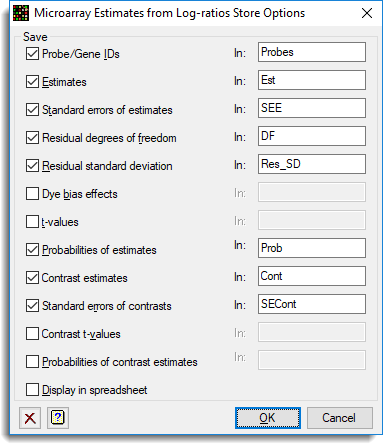1. Home
2. Store Microarray Estimates from Log-ratios

# Store Microarray Estimates from Log-ratios

Use this to save results when estimating log-ratios for microarray data. These results are saved when an analysis is run and must be specified before running an analysis.

1. After selecting the appropriate boxes, type names for the data structures into the corresponding In fields.## Save

The table below indicates the type of structures formed for each item.

 Probe/Gene IDs Factor The list of probes/gene identifiers to index the results. Estimates Pointer The estimates will be saved in unnamed variates pointed to by this pointer. There will be one variate for each treatment/target. Standard errors of estimates Pointer The standard errors of the estimates will be saved in unnamed variates pointed to by this pointer. There will be one variate for each treatment/target. Residual degrees of freedom Variate The residual degrees of freedom from the ANOVA for each probe/gene. Residual standard deviation Variate The square root of the residual mean square from the ANOVA for each probe/gene. Dye bias effects Variate The estimated dye bias effects for each probe/gene. t-values Pointer The t-values (the estimates divided by their standard errors) will be saved in unnamed variates pointed to by this pointer. There will be one variate for each treatment/target. Probabilities of estimates Pointer The two sided probabilities of a t-value of the estimates being more extreme than the observed t-values will be saved in unnamed variates pointed to by this pointer. There will be one variate for each treatment/target. Contrast estimates Pointer The contrast estimates will be saved in unnamed variates pointed to by this pointer. There will be one variate for each contrast. Contrast t-values Pointer The contrast t-values (the contrasts divided by their standard errors) will be saved in unnamed variates pointed to by this pointer. There will be one variate for each contrast. Probabilities of contrast estimates Pointer The two sided probabilities of contrast t-values being more extreme than the observed t-values will be saved in unnamed variates pointed to by this pointer. There will be one variate for each contrast.Clear Clear all fields and list boxes.Help Open the Help topic for this dialog.